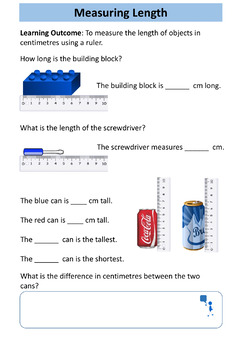# Length and Height: Special Education MathSubject
Resource Type
File Type

Zip

(5 MB|22 pages)
Product Rating
Standards
Also included in:
1. These workbooks have been designed for older Special Education students who are working at Kindergarten / Grade 1 level.This bundle contains 10 highly visual and age-appropriate workbooks/worksheets with answers, progress checks and certificates of achievement. In total there are 205 worksheets that
\$39.90
\$24.99
Save \$14.91
• Product Description
• StandardsNEW

This math resource has been designed for Special Education students. It contains an age-appropriate, 22-page workbook (22 worksheets) with progress check, certificate of achievement and answers on length and height.

• Comparing lengths and heights
• Using the words long, longer, longest, short, shorter, shortest, tall, taller, tallest to describe length and height
• Calculating the length of a line drawn on a centimeter grid
• Length and height-related reasoning and problem-solving

You may also be interested in:

Volume and Capacity Workbook - Special Education Math

• Comparing the volume of containers
• Comparing the capacity of containers
• Describing volume using the key words empty, half full, full
• Applying knowledge of volume and capacity to solve simple problems

You may also be interested in:

Time: Special Education Workbook

• Naming the days of the week and put them in order.
• Reading the time to the hour on an
• Drawing the hands to show o’clock
• Telling the time to the half-hour
• Drawing the hands to show half past
• Using the terms - today, yesterday, tomorrow
• Solving simple time problems

Fractions Halves: Special Education Workbook

This contains a 25-page workbook (25 worksheets) with progress check, certificate of achievement and answers on halves of quantities, amounts and numbers up to 20:

• Using the = sign
• Identifying halves of shapes
• Identifying halves of amounts
• Calculating halves of even numbers to 20
• Applying knowledge of halves to solve simple number and word problems

Addition and Subtraction to 20 Workbook: Special Education Math

This is a 36-page workbook (36 worksheets) with progress check, certificate of achievement and answers for addition and subtraction to 20.

• Adding two whole numbers with a total up to 20
• Subtracting one number up to 20 from another
• Using addition and subtraction to solve simple number problems
• Understanding that subtraction is the inverse of addition

Counting to 20 Workbook: Special Education Math

This is a 20-page workbook (20 worksheets) with progress check, certificate of achievement and answers on number recognition and counting to 20.

• Counting up to 20 items
• Reading and writing numbers up to 20
• Ordering and comparing numbers up to 20
• Completing a number line up to 20
• Solving simple word problems involving numbers up to 20

Number Recognition and Counting to 20 PowerPoint Lesson

This is an age-appropriate 130-slide PowerPoint lesson to accompany the workbook/worksheets.

Save money and buy both resources in a bundle at a discounted rate:

Number Recognition and Counting to 20 PowerPoint Lesson and Workbook / Worksheets

Addition and Subtraction to 20 Workbook

This resource contains a 36-page workbook (36 worksheets) with progress check, certificate of achievement and answers for addition and subtraction to 20.

• Adding two whole numbers with a total up to 20
• Subtracting one number up to 20 from another
• Using addition and subtraction to solve simple number problems
• Understanding that subtraction is the inverse of addition

More Special Education Math Resources

Keep informed of new resources by following me here.

Express the length of an object as a whole number of length units, by laying multiple copies of a shorter object (the length unit) end to end; understand that the length measurement of an object is the number of same-size length units that span it with no gaps or overlaps.
Order three objects by length; compare the lengths of two objects indirectly by using a third object.
Directly compare two objects with a measurable attribute in common, to see which object has “more of”/“less of” the attribute, and describe the difference. For example, directly compare the heights of two children and describe one child as taller/shorter.
Describe measurable attributes of objects, such as length or weight. Describe several measurable attributes of a single object.
Total Pages
22 pages
Included
Teaching Duration
N/A
Report this Resource to TpT
Reported resources will be reviewed by our team. Report this resource to let us know if this resource violates TpT’s content guidelines.